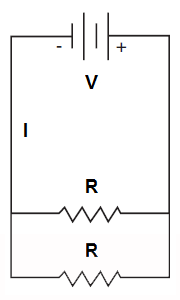## Electronics Information Flash Card Set 623012

Card 1 of 10
###### Parallel Circuit

In a parallel circuit, each load occupies a separate parallel path in the circuit and the input voltage is fully applied to each path. Unlike a series circuit where current (I) is the same at all points in the circuit, in a parallel circuit, voltage (V) is the same across each parallel branch of the circuit but current differs in each branch depending on the load (resistance) present.

##### parallelThis is an example of a(n) __________ circuit.